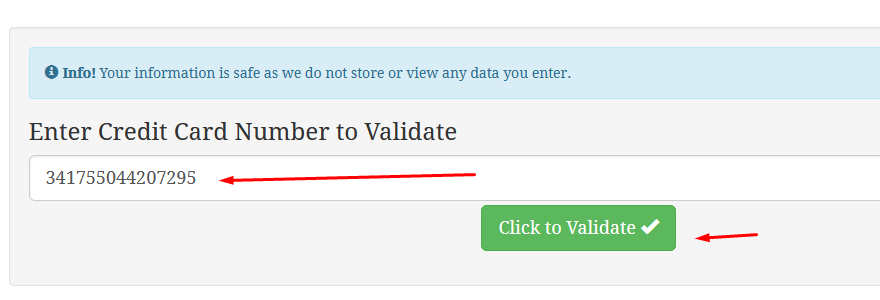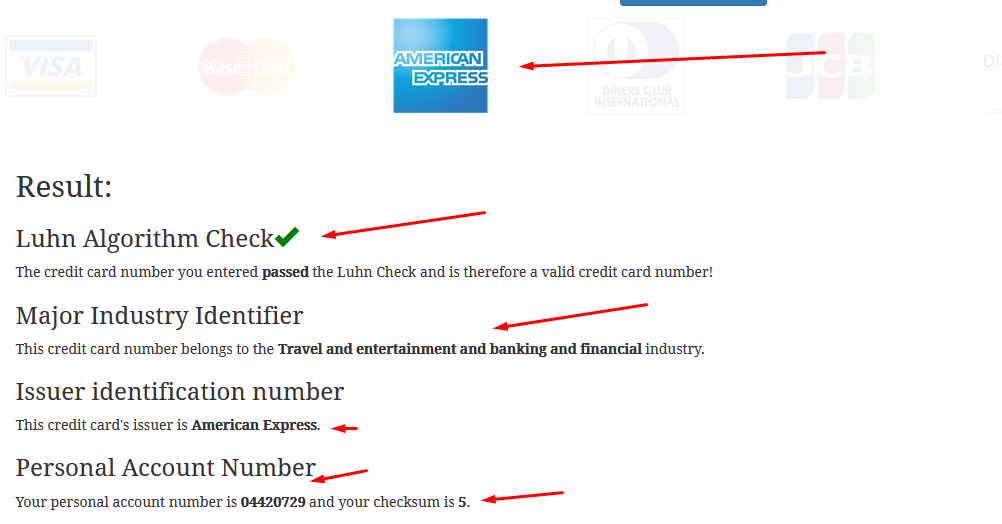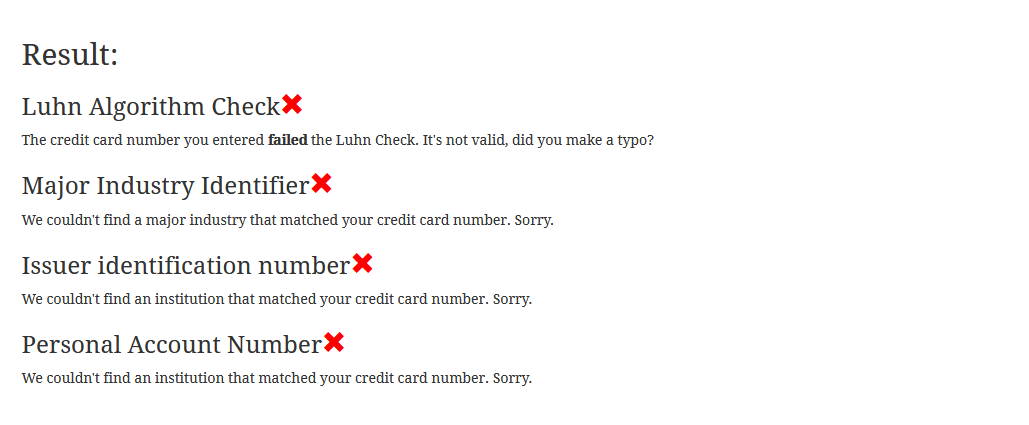# How to Test Credit Card Numbers!## Also checkout our new tool IBAN Checker

For premium API access refer to our

So you got a credit card number yet do not know if its really a valid credit card number. Note that a valid credit card number are not just created randomly. It has a formula to generate a credit card number.

## Validated a credit card number

Here's an easy and quick way to check the credit card number if its valid or not. One way is to use credit card checkers or validators. At ccardgenerator.com we offer a quick and easy way to check credit card numbers for validity.

• Simply to go Credit card validator page.
• Enter the credit card number you want to validate.
• Hit the "click to validate" button.
•• In an instant you will get your result.
• ### Valid Credit Card number

• You will know the credit card issuer, the personal account number, and if its checksum valid.
•### Invalid Credit Card number

• If you enter an invalid credit card number here's the result
•### How we check credit card number?

To check if the credit card number is valid. We check for the following:

• First Digit - Major Industry Identifier
• Six Digit - Issuer identification number
• Seven Digit - Personal Account Number and Checksum

The check digit (x) is obtained by computing the sum of the non-check digits then computing 9 times that value modulo 10 (in equation form, ((67 × 9) mod 10)). In algorithm form:

• Compute the sum of the non-check digits (67).
• Multiply by 9 (603).
• The units digit (3) is the check digit. Thus, x=3.

(Alternative method) The check digit (x) is obtained by computing the sum of the other digits (third row) then subtracting the units digit from 10 (67 => Units digit 7; 10 − 7 = check digit 3). In algorithm form:

• Compute the sum of the non-check digits (67).
• Take the units digit (7).
• Subtract the units digit from 10.
• The result (3) is the check digit. In case the sum of digits ends in 0 then 0 is the check digit.

INFO: Credit card number generated are valid but DOES NOT WORK like an actual credit card. They do not have any actual REAL VALUE. They are for data testing and verification purposes only.